## Neural Network

### Using MNIST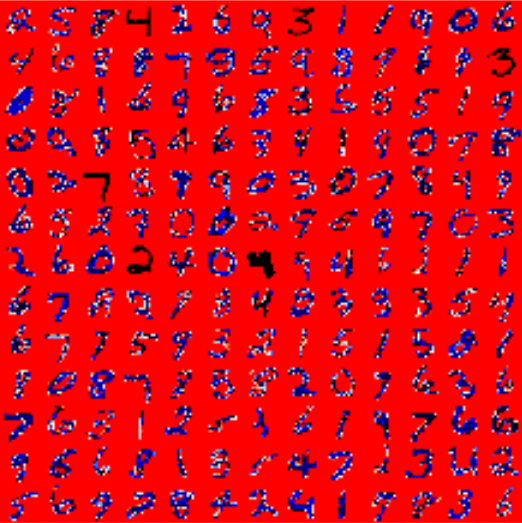The MNIST database (Modified National Institute of Standards and Technology database) of handwritten digits consists of a training set of 60,000 examples, and a test set of 10,000 examples. It is a subset of a larger set available from NIST. Additionally, the black and white images from NIST were size-normalized and centered to fit into a 28x28 pixel bounding box and anti-aliased, which introduced grayscale levels.

This database is well liked for training and testing in the field of machine learning and image processing. It is a remixed subset of the original NIST datasets. One half of the 60,000 training images consist of images from NIST's testing dataset and the other half from Nist's training set. The 10,000 images from the testing set are similarly assembled.

The MNIST dataset is used by researchers to test and compare their research results with others. The lowest error rates in literature are as low as 0.21 percent.1

### Reading the MNIST data set

The images from the data set have the size 28 x 28. They are saved in the csv data files mnist_train.csv and mnist_test.csv.

Every line of these files consists of an image, i.e. 785 numbers between 0 and 255.

The first number of each line is the label, i.e. the digit which is depicted in the image. The following 784 numbers are the pixels of the 28 x 28 image.

%matplotlib inline
import numpy as np
import matplotlib.pyplot as plt

image_size = 28 # width and length
no_of_different_labels = 10 #  i.e. 0, 1, 2, 3, ..., 9
image_pixels = image_size * image_size
data_path = "data/mnist/"
delimiter=",")
delimiter=",")
test_data[:10]

Output::
array([[7., 0., 0., ..., 0., 0., 0.],
[2., 0., 0., ..., 0., 0., 0.],
[1., 0., 0., ..., 0., 0., 0.],
...,
[9., 0., 0., ..., 0., 0., 0.],
[5., 0., 0., ..., 0., 0., 0.],
[9., 0., 0., ..., 0., 0., 0.]])
test_data[test_data==255]
test_data.shape

Output::
(10000, 785)

The images of the MNIST dataset are greyscale and the pixels range between 0 and 255 including both bounding values. We will map these values into an interval from [0.01, 1] by multiplying each pixel by 0.99 / 255 and adding 0.01 to the result. This way, we avoid 0 values as inputs, which are capable of preventing weight updates, as we we seen in the introductory chapter.

fac = 0.99 / 255
train_imgs = np.asfarray(train_data[:, 1:]) * fac + 0.01
test_imgs = np.asfarray(test_data[:, 1:]) * fac + 0.01

train_labels = np.asfarray(train_data[:, :1])
test_labels = np.asfarray(test_data[:, :1])


We need the labels in our calculations in a one-hot representation. We have 10 digits from 0 to 9, i.e. lr = np.arange(10).

Turning a label into one-hot representation can be achieved with the command: (lr==label).astype(np.int)

We demonstrate this in the following:

import numpy as np

lr = np.arange(10)

for label in range(10):
one_hot = (lr==label).astype(np.int)
print("label: ", label, " in one-hot representation: ", one_hot)

label:  0  in one-hot representation:  [1 0 0 0 0 0 0 0 0 0]
label:  1  in one-hot representation:  [0 1 0 0 0 0 0 0 0 0]
label:  2  in one-hot representation:  [0 0 1 0 0 0 0 0 0 0]
label:  3  in one-hot representation:  [0 0 0 1 0 0 0 0 0 0]
label:  4  in one-hot representation:  [0 0 0 0 1 0 0 0 0 0]
label:  5  in one-hot representation:  [0 0 0 0 0 1 0 0 0 0]
label:  6  in one-hot representation:  [0 0 0 0 0 0 1 0 0 0]
label:  7  in one-hot representation:  [0 0 0 0 0 0 0 1 0 0]
label:  8  in one-hot representation:  [0 0 0 0 0 0 0 0 1 0]
label:  9  in one-hot representation:  [0 0 0 0 0 0 0 0 0 1]


We are ready now to turn our labelled images into one-hot representations. Instead of zeroes and one, we create 0.01 and 0.99, which will be better for our calculations:

lr = np.arange(no_of_different_labels)

# transform labels into one hot representation
train_labels_one_hot = (lr==train_labels).astype(np.float)
test_labels_one_hot = (lr==test_labels).astype(np.float)

# we don't want zeroes and ones in the labels neither:
train_labels_one_hot[train_labels_one_hot==0] = 0.01
train_labels_one_hot[train_labels_one_hot==1] = 0.99
test_labels_one_hot[test_labels_one_hot==0] = 0.01
test_labels_one_hot[test_labels_one_hot==1] = 0.99


Before we start using the MNIST data sets with our neural network, we will have a look at some images:

for i in range(10):
img = train_imgs[i].reshape((28,28))
plt.imshow(img, cmap="Greys")
plt.show()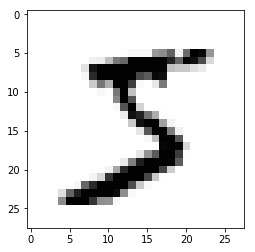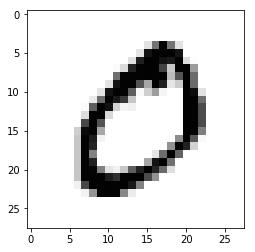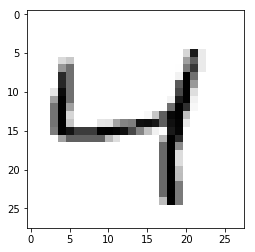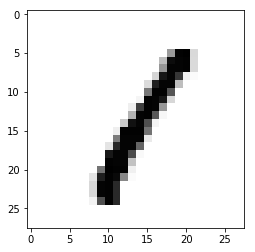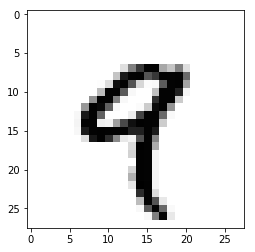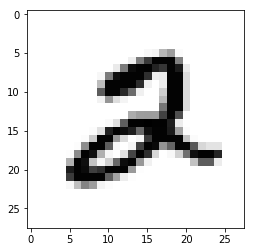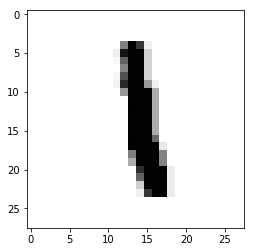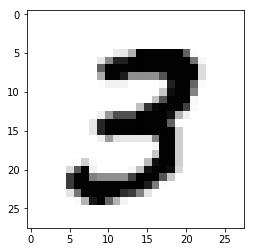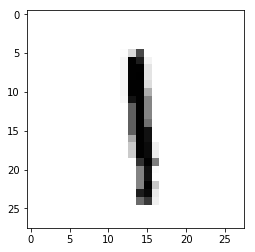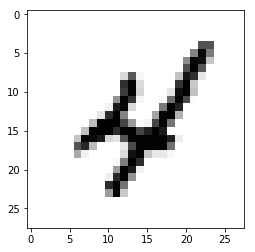### Dumping the Data for Faster Reload

You may have noticed that it is quite slow to read in the data from the csv files.

We will save the data in binary format with the dump function from the pickle module:

import pickle

with open("data/mnist/pickled_mnist.pkl", "bw") as fh:
data = (train_imgs,
test_imgs,
train_labels,
test_labels,
train_labels_one_hot,
test_labels_one_hot)
pickle.dump(data, fh)



We are able now to read in the data by using pickle.load. This is a lot faster than using loadtxt on the csv files:

import pickle

with open("data/mnist/pickled_mnist.pkl", "br") as fh:

train_imgs = data
test_imgs = data
train_labels = data
test_labels = data
train_labels_one_hot = data
test_labels_one_hot = data

image_size = 28 # width and length
no_of_different_labels = 10 #  i.e. 0, 1, 2, 3, ..., 9
image_pixels = image_size * image_size


### Classifying the Data

We will use the following neuronal network class for our first classification:

import numpy as np

@np.vectorize
def sigmoid(x):
return 1 / (1 + np.e ** -x)
activation_function = sigmoid

from scipy.stats import truncnorm

def truncated_normal(mean=0, sd=1, low=0, upp=10):
return truncnorm((low - mean) / sd,
(upp - mean) / sd,
loc=mean,
scale=sd)

class NeuralNetwork:

def __init__(self,
no_of_in_nodes,
no_of_out_nodes,
no_of_hidden_nodes,
learning_rate):
self.no_of_in_nodes = no_of_in_nodes
self.no_of_out_nodes = no_of_out_nodes
self.no_of_hidden_nodes = no_of_hidden_nodes
self.learning_rate = learning_rate
self.create_weight_matrices()

def create_weight_matrices(self):
"""
A method to initialize the weight
matrices of the neural network
"""
X = truncated_normal(mean=0,
sd=1,
self.wih = X.rvs((self.no_of_hidden_nodes,
self.no_of_in_nodes))
self.who = X.rvs((self.no_of_out_nodes,
self.no_of_hidden_nodes))

def train(self, input_vector, target_vector):
"""
input_vector and target_vector can
be tuple, list or ndarray
"""

input_vector = np.array(input_vector, ndmin=2).T
target_vector = np.array(target_vector, ndmin=2).T

output_vector1 = np.dot(self.wih,
input_vector)
output_hidden = activation_function(output_vector1)

output_vector2 = np.dot(self.who,
output_hidden)
output_network = activation_function(output_vector2)

output_errors = target_vector - output_network
# update the weights:
tmp = output_errors * output_network \
* (1.0 - output_network)
tmp = self.learning_rate  * np.dot(tmp,
output_hidden.T)
self.who += tmp

# calculate hidden errors:
hidden_errors = np.dot(self.who.T,
output_errors)
# update the weights:
tmp = hidden_errors * output_hidden * \
(1.0 - output_hidden)
self.wih += self.learning_rate \
* np.dot(tmp, input_vector.T)

def run(self, input_vector):
# input_vector can be tuple, list or ndarray
input_vector = np.array(input_vector, ndmin=2).T

output_vector = np.dot(self.wih,
input_vector)
output_vector = activation_function(output_vector)

output_vector = np.dot(self.who,
output_vector)
output_vector = activation_function(output_vector)

return output_vector

def confusion_matrix(self, data_array, labels):
cm = np.zeros((10, 10), int)
for i in range(len(data_array)):
res = self.run(data_array[i])
res_max = res.argmax()
target = labels[i]
cm[res_max, int(target)] += 1
return cm

def precision(self, label, confusion_matrix):
col = confusion_matrix[:, label]
return confusion_matrix[label, label] / col.sum()

def recall(self, label, confusion_matrix):
row = confusion_matrix[label, :]
return confusion_matrix[label, label] / row.sum()

def evaluate(self, data, labels):
corrects, wrongs = 0, 0
for i in range(len(data)):
res = self.run(data[i])
res_max = res.argmax()
if res_max == labels[i]:
corrects += 1
else:
wrongs += 1
return corrects, wrongs


ANN = NeuralNetwork(no_of_in_nodes = image_pixels,
no_of_out_nodes = 10,
no_of_hidden_nodes = 100,
learning_rate = 0.1)

for i in range(len(train_imgs)):
ANN.train(train_imgs[i], train_labels_one_hot[i])

for i in range(20):
res = ANN.run(test_imgs[i])
print(test_labels[i], np.argmax(res), np.max(res))


[7.] 7 0.9829245583409039
[2.] 2 0.7372766887508578
[1.] 1 0.9881823673106839
[0.] 0 0.9873289971465894
[4.] 4 0.9456335245615916
[1.] 1 0.9880120617106172
[4.] 4 0.976550583573903
[9.] 9 0.964909168118122
[5.] 6 0.36615932726182665
[9.] 9 0.9848677489827125
[0.] 0 0.9204097234781773
[6.] 6 0.8897871402453337
[9.] 9 0.9936811621891628
[0.] 0 0.9832119513084644
[1.] 1 0.988750833073612
[5.] 5 0.9156741221523511
[9.] 9 0.9812577974620423
[7.] 7 0.9888560485875889
[3.] 3 0.8772868556722897
[4.] 4 0.9900030761222965

corrects, wrongs = ANN.evaluate(train_imgs, train_labels)
print("accuracy train: ", corrects / ( corrects + wrongs))
corrects, wrongs = ANN.evaluate(test_imgs, test_labels)
print("accuracy: test", corrects / ( corrects + wrongs))

cm = ANN.confusion_matrix(train_imgs, train_labels)
print(cm)

for i in range(10):
print("digit: ", i, "precision: ", ANN.precision(i, cm), "recall: ", ANN.recall(i, cm))

accuracy train:  0.9469166666666666
accuracy: test 0.9459
[[5802    0   53   21    9   42   35    8   14   20]
[   1 6620   45   22    6   29   14   50   75    7]
[   5   22 5486   51   10   11    5   53   11    3]
[   6   36  114 5788    2  114    1   35   76   72]
[   8   16   54    8 5439   41   10   52   25   90]
[   5    2    3   44    0 4922   20    3    5   11]
[  37    4   54   19   71   72 5789    3   41    4]
[   0    5   31   38    7    4    0 5762    1   32]
[  52   20  103   83    9  102   43   21 5535   38]
[   7   17   15   57  289   84    1  278   68 5672]]
digit:  0 precision:  0.9795711632618606 recall:  0.9663557628247835
digit:  1 precision:  0.9819044793829724 recall:  0.9637501819769981
digit:  2 precision:  0.9207787848271232 recall:  0.9697719639384833
digit:  3 precision:  0.9440548034578372 recall:  0.9269698910954516
digit:  4 precision:  0.9310167750770284 recall:  0.9470659933832491
digit:  5 precision:  0.9079505626268216 recall:  0.9814556331006979
digit:  6 precision:  0.978202095302467 recall:  0.9499507712504103
digit:  7 precision:  0.9197126895450918 recall:  0.9799319727891157
digit:  8 precision:  0.945992138096052 recall:  0.9215784215784216
digit:  9 precision:  0.953437552529837 recall:  0.87422934648582


## Multiple Runs

We can repeat the training multiple times. Each run is called an "epoch".

epochs = 3

NN = NeuralNetwork(no_of_in_nodes = image_pixels,
no_of_out_nodes = 10,
no_of_hidden_nodes = 100,
learning_rate = 0.1)

for epoch in range(epochs):
print("epoch: ", epoch)
for i in range(len(train_imgs)):
NN.train(train_imgs[i],
train_labels_one_hot[i])

corrects, wrongs = NN.evaluate(train_imgs, train_labels)
print("accuracy train: ", corrects / ( corrects + wrongs))
corrects, wrongs = NN.evaluate(test_imgs, test_labels)
print("accuracy: test", corrects / ( corrects + wrongs))

epoch:  0
accruracy train:  0.94515
accruracy: test 0.9459
epoch:  1
accruracy train:  0.9626833333333333
accruracy: test 0.9582
epoch:  2
accruracy train:  0.96995
accruracy: test 0.9626


We want to do the multiple training of the training set inside of our network. To this purpose we rewrite the method train and add a method train_single. train_single is more or less what we called 'train' before. Whereas the new 'train' method is doing the epoch counting. For testing purposes, we save the weight matrices after each epoch in
the list intermediate_weights. This list is returned as the output of train:

import numpy as np

@np.vectorize
def sigmoid(x):
return 1 / (1 + np.e ** -x)
activation_function = sigmoid

from scipy.stats import truncnorm

def truncated_normal(mean=0, sd=1, low=0, upp=10):
return truncnorm((low - mean) / sd,
(upp - mean) / sd,
loc=mean,
scale=sd)

class NeuralNetwork:

def __init__(self,
no_of_in_nodes,
no_of_out_nodes,
no_of_hidden_nodes,
learning_rate):
self.no_of_in_nodes = no_of_in_nodes
self.no_of_out_nodes = no_of_out_nodes
self.no_of_hidden_nodes = no_of_hidden_nodes
self.learning_rate = learning_rate
self.create_weight_matrices()

def create_weight_matrices(self):
""" A method to initialize the weight matrices of the neural network"""
X = truncated_normal(mean=0,
sd=1,
self.wih = X.rvs((self.no_of_hidden_nodes,
self.no_of_in_nodes))
X = truncated_normal(mean=0,
sd=1,
self.who = X.rvs((self.no_of_out_nodes,
self.no_of_hidden_nodes))

def train_single(self, input_vector, target_vector):
"""
input_vector and target_vector can be tuple,
list or ndarray
"""

output_vectors = []
input_vector = np.array(input_vector, ndmin=2).T
target_vector = np.array(target_vector, ndmin=2).T

output_vector1 = np.dot(self.wih,
input_vector)
output_hidden = activation_function(output_vector1)

output_vector2 = np.dot(self.who,
output_hidden)
output_network = activation_function(output_vector2)

output_errors = target_vector - output_network
# update the weights:
tmp = output_errors * output_network * \
(1.0 - output_network)
tmp = self.learning_rate  * np.dot(tmp,
output_hidden.T)
self.who += tmp

# calculate hidden errors:
hidden_errors = np.dot(self.who.T,
output_errors)
# update the weights:
tmp = hidden_errors * output_hidden * (1.0 - output_hidden)
self.wih += self.learning_rate * np.dot(tmp, input_vector.T)

def train(self, data_array,
labels_one_hot_array,
epochs=1,
intermediate_results=False):
intermediate_weights = []
for epoch in range(epochs):
print("*", end="")
for i in range(len(data_array)):
self.train_single(data_array[i],
labels_one_hot_array[i])
if intermediate_results:
intermediate_weights.append((self.wih.copy(),
self.who.copy()))
return intermediate_weights

def confusion_matrix(self, data_array, labels):
cm = {}
for i in range(len(data_array)):
res = self.run(data_array[i])
res_max = res.argmax()
target = labels[i]
if (target, res_max) in cm:
cm[(target, res_max)] += 1
else:
cm[(target, res_max)] = 1
return cm

def run(self, input_vector):
""" input_vector can be tuple, list or ndarray """

input_vector = np.array(input_vector, ndmin=2).T

output_vector = np.dot(self.wih,
input_vector)
output_vector = activation_function(output_vector)

output_vector = np.dot(self.who,
output_vector)
output_vector = activation_function(output_vector)

return output_vector

def evaluate(self, data, labels):
corrects, wrongs = 0, 0
for i in range(len(data)):
res = self.run(data[i])
res_max = res.argmax()
if res_max == labels[i]:
corrects += 1
else:
wrongs += 1
return corrects, wrongs


epochs = 10

ANN = NeuralNetwork(no_of_in_nodes = image_pixels,
no_of_out_nodes = 10,
no_of_hidden_nodes = 100,
learning_rate = 0.15)

weights = ANN.train(train_imgs,
train_labels_one_hot,
epochs=epochs,
intermediate_results=True)


**********
cm = ANN.confusion_matrix(train_imgs, train_labels)

print(ANN.run(train_imgs[i]))

[[2.60149245e-03]
[2.52542556e-03]
[6.57990628e-03]
[1.32663729e-03]
[1.34985384e-03]
[2.63840265e-04]
[2.18329159e-04]
[1.32693720e-04]
[9.84326084e-01]
[4.34559417e-02]]

cm = list(cm.items())
print(sorted(cm))

[((0.0, 0), 5853), ((0.0, 1), 1), ((0.0, 2), 3), ((0.0, 4), 8), ((0.0, 5), 2), ((0.0, 6), 12), ((0.0, 7), 7), ((0.0, 8), 27), ((0.0, 9), 10), ((1.0, 0), 1), ((1.0, 1), 6674), ((1.0, 2), 17), ((1.0, 3), 5), ((1.0, 4), 14), ((1.0, 5), 2), ((1.0, 6), 1), ((1.0, 7), 6), ((1.0, 8), 15), ((1.0, 9), 7), ((2.0, 0), 37), ((2.0, 1), 14), ((2.0, 2), 5791), ((2.0, 3), 17), ((2.0, 4), 11), ((2.0, 5), 2), ((2.0, 6), 10), ((2.0, 7), 15), ((2.0, 8), 51), ((2.0, 9), 10), ((3.0, 0), 16), ((3.0, 1), 5), ((3.0, 2), 34), ((3.0, 3), 5869), ((3.0, 4), 8), ((3.0, 5), 57), ((3.0, 6), 4), ((3.0, 7), 20), ((3.0, 8), 58), ((3.0, 9), 60), ((4.0, 0), 14), ((4.0, 1), 6), ((4.0, 2), 8), ((4.0, 3), 1), ((4.0, 4), 5678), ((4.0, 5), 1), ((4.0, 6), 14), ((4.0, 7), 5), ((4.0, 8), 11), ((4.0, 9), 104), ((5.0, 0), 7), ((5.0, 1), 2), ((5.0, 2), 6), ((5.0, 3), 27), ((5.0, 4), 5), ((5.0, 5), 5312), ((5.0, 6), 12), ((5.0, 7), 5), ((5.0, 8), 20), ((5.0, 9), 25), ((6.0, 0), 32), ((6.0, 1), 5), ((6.0, 2), 1), ((6.0, 4), 10), ((6.0, 5), 52), ((6.0, 6), 5791), ((6.0, 8), 26), ((6.0, 9), 1), ((7.0, 0), 5), ((7.0, 1), 11), ((7.0, 2), 22), ((7.0, 3), 2), ((7.0, 4), 17), ((7.0, 5), 3), ((7.0, 6), 2), ((7.0, 7), 6074), ((7.0, 8), 26), ((7.0, 9), 103), ((8.0, 0), 20), ((8.0, 1), 18), ((8.0, 2), 9), ((8.0, 3), 14), ((8.0, 4), 27), ((8.0, 5), 24), ((8.0, 6), 9), ((8.0, 7), 8), ((8.0, 8), 5668), ((8.0, 9), 54), ((9.0, 0), 26), ((9.0, 1), 2), ((9.0, 2), 2), ((9.0, 3), 16), ((9.0, 4), 69), ((9.0, 5), 14), ((9.0, 6), 7), ((9.0, 7), 19), ((9.0, 8), 15), ((9.0, 9), 5779)]

In [ ]:
for i in range(epochs):
print("epoch: ", i)
ANN.wih = weights[i]
ANN.who = weights[i]

corrects, wrongs = ANN.evaluate(train_imgs, train_labels)
print("accuracy train: ", corrects / ( corrects + wrongs))
corrects, wrongs = ANN.evaluate(test_imgs, test_labels)
print("accuracy: test", corrects / ( corrects + wrongs))


## With Bias Nodes

import numpy as np

@np.vectorize
def sigmoid(x):
return 1 / (1 + np.e ** -x)
activation_function = sigmoid

from scipy.stats import truncnorm

def truncated_normal(mean=0, sd=1, low=0, upp=10):
return truncnorm((low - mean) / sd,
(upp - mean) / sd,
loc=mean,
scale=sd)

class NeuralNetwork:

def __init__(self,
no_of_in_nodes,
no_of_out_nodes,
no_of_hidden_nodes,
learning_rate,
bias=None
):

self.no_of_in_nodes = no_of_in_nodes
self.no_of_out_nodes = no_of_out_nodes
self.no_of_hidden_nodes = no_of_hidden_nodes
self.learning_rate = learning_rate
self.bias = bias
self.create_weight_matrices()

def create_weight_matrices(self):
"""
A method to initialize the weight
matrices of the neural network with
optional bias nodes
"""

bias_node = 1 if self.bias else 0

rad = 1 / np.sqrt(self.no_of_in_nodes + bias_node)
X = truncated_normal(mean=0,
sd=1,
self.wih = X.rvs((self.no_of_hidden_nodes,
self.no_of_in_nodes + bias_node))

rad = 1 / np.sqrt(self.no_of_hidden_nodes + bias_node)
self.who = X.rvs((self.no_of_out_nodes,
self.no_of_hidden_nodes + bias_node))

def train(self, input_vector, target_vector):
"""
input_vector and target_vector can
be tuple, list or ndarray
"""

bias_node = 1 if self.bias else 0
if self.bias:
# adding bias node to the end of the inpuy_vector
input_vector = np.concatenate((input_vector,
[self.bias]) )

input_vector = np.array(input_vector, ndmin=2).T
target_vector = np.array(target_vector, ndmin=2).T

output_vector1 = np.dot(self.wih,
input_vector)
output_hidden = activation_function(output_vector1)

if self.bias:
output_hidden = np.concatenate((output_hidden,
[[self.bias]]) )

output_vector2 = np.dot(self.who,
output_hidden)
output_network = activation_function(output_vector2)

output_errors = target_vector - output_network
# update the weights:
tmp = output_errors * output_network * (1.0 - output_network)
tmp = self.learning_rate  * np.dot(tmp, output_hidden.T)
self.who += tmp

# calculate hidden errors:
hidden_errors = np.dot(self.who.T,
output_errors)
# update the weights:
tmp = hidden_errors * output_hidden * (1.0 - output_hidden)
if self.bias:
x = np.dot(tmp, input_vector.T)[:-1,:]
else:
x = np.dot(tmp, input_vector.T)
self.wih += self.learning_rate * x

def run(self, input_vector):
"""
input_vector can be tuple, list or ndarray
"""

if self.bias:
# adding bias node to the end of the inpuy_vector
input_vector = np.concatenate((input_vector, ) )
input_vector = np.array(input_vector, ndmin=2).T

output_vector = np.dot(self.wih,
input_vector)
output_vector = activation_function(output_vector)

if self.bias:
output_vector = np.concatenate( (output_vector,
[]) )

output_vector = np.dot(self.who,
output_vector)
output_vector = activation_function(output_vector)
return output_vector

def evaluate(self, data, labels):
corrects, wrongs = 0, 0
for i in range(len(data)):
res = self.run(data[i])
res_max = res.argmax()
if res_max == labels[i]:
corrects += 1
else:
wrongs += 1
return corrects, wrongs


ANN = NeuralNetwork(no_of_in_nodes=image_pixels,
no_of_out_nodes=10,
no_of_hidden_nodes=200,
learning_rate=0.1,
bias=None)

for i in range(len(train_imgs)):
ANN.train(train_imgs[i], train_labels_one_hot[i])
for i in range(20):
res = ANN.run(test_imgs[i])
print(test_labels[i], np.argmax(res), np.max(res))

[7.] 7 0.9951478957895473
[2.] 2 0.9167137305226186
[1.] 1 0.9930670538508068
[0.] 0 0.9729093609525741
[4.] 4 0.9475097483176407
[1.] 1 0.9919906877733081
[4.] 4 0.9390079959736829
[9.] 9 0.9815469745110644
[5.] 5 0.23871278844097427
[9.] 9 0.9863859218561386
[0.] 0 0.9667234471027278
[6.] 6 0.8856024953669486
[9.] 9 0.9928943830319253
[0.] 0 0.96922568081586
[1.] 1 0.9899747475376088
[5.] 5 0.9595147911735664
[9.] 9 0.9958119066147573
[7.] 7 0.9883146384365381
[3.] 3 0.8706223167904136
[4.] 4 0.9912284156702522

corrects, wrongs = ANN.evaluate(train_imgs, train_labels)
print("accuracy train: ", corrects / ( corrects + wrongs))
corrects, wrongs = ANN.evaluate(test_imgs, test_labels)
print("accuracy: test", corrects / ( corrects + wrongs))

accruracy train:  0.9555666666666667
accruracy: test 0.9544


### Version with Bias and Epochs:

import numpy as np

@np.vectorize
def sigmoid(x):
return 1 / (1 + np.e ** -x)
activation_function = sigmoid

from scipy.stats import truncnorm

def truncated_normal(mean=0, sd=1, low=0, upp=10):
return truncnorm((low - mean) / sd,
(upp - mean) / sd,
loc=mean,
scale=sd)

class NeuralNetwork:

def __init__(self,
no_of_in_nodes,
no_of_out_nodes,
no_of_hidden_nodes,
learning_rate,
bias=None
):

self.no_of_in_nodes = no_of_in_nodes
self.no_of_out_nodes = no_of_out_nodes

self.no_of_hidden_nodes = no_of_hidden_nodes

self.learning_rate = learning_rate
self.bias = bias
self.create_weight_matrices()

def create_weight_matrices(self):
"""
A method to initialize the weight matrices
of the neural network with optional
bias nodes"""

bias_node = 1 if self.bias else 0

rad = 1 / np.sqrt(self.no_of_in_nodes + bias_node)
self.wih = X.rvs((self.no_of_hidden_nodes,
self.no_of_in_nodes + bias_node))

rad = 1 / np.sqrt(self.no_of_hidden_nodes + bias_node)
X = truncated_normal(mean=0,
sd=1,
self.who = X.rvs((self.no_of_out_nodes,
self.no_of_hidden_nodes + bias_node))

def train_single(self, input_vector, target_vector):
"""
input_vector and target_vector can be tuple,
list or ndarray
"""

bias_node = 1 if self.bias else 0
if self.bias:
# adding bias node to the end of the inpuy_vector
input_vector = np.concatenate( (input_vector,
[self.bias]) )

output_vectors = []
input_vector = np.array(input_vector, ndmin=2).T
target_vector = np.array(target_vector, ndmin=2).T

output_vector1 = np.dot(self.wih,
input_vector)
output_hidden = activation_function(output_vector1)

if self.bias:
output_hidden = np.concatenate((output_hidden,
[[self.bias]]) )

output_vector2 = np.dot(self.who,
output_hidden)
output_network = activation_function(output_vector2)

output_errors = target_vector - output_network
# update the weights:
tmp = output_errors * output_network * (1.0 - output_network)
tmp = self.learning_rate  * np.dot(tmp,
output_hidden.T)
self.who += tmp

# calculate hidden errors:
hidden_errors = np.dot(self.who.T,
output_errors)
# update the weights:
tmp = hidden_errors * output_hidden * (1.0 - output_hidden)
if self.bias:
x = np.dot(tmp, input_vector.T)[:-1,:]
else:
x = np.dot(tmp, input_vector.T)
self.wih += self.learning_rate * x

def train(self, data_array,
labels_one_hot_array,
epochs=1,
intermediate_results=False):
intermediate_weights = []
for epoch in range(epochs):
for i in range(len(data_array)):
self.train_single(data_array[i],
labels_one_hot_array[i])
if intermediate_results:
intermediate_weights.append((self.wih.copy(),
self.who.copy()))
return intermediate_weights

def run(self, input_vector):
# input_vector can be tuple, list or ndarray

if self.bias:
# adding bias node to the end of the inpuy_vector
input_vector = np.concatenate( (input_vector,
[self.bias]) )
input_vector = np.array(input_vector, ndmin=2).T

output_vector = np.dot(self.wih,
input_vector)
output_vector = activation_function(output_vector)

if self.bias:
output_vector = np.concatenate( (output_vector,
[[self.bias]]) )

output_vector = np.dot(self.who,
output_vector)
output_vector = activation_function(output_vector)

return output_vector

def evaluate(self, data, labels):
corrects, wrongs = 0, 0
for i in range(len(data)):
res = self.run(data[i])
res_max = res.argmax()
if res_max == labels[i]:
corrects += 1
else:
wrongs += 1
return corrects, wrongs


epochs = 12

network = NeuralNetwork(no_of_in_nodes=image_pixels,
no_of_out_nodes=10,
no_of_hidden_nodes=100,
learning_rate=0.1,
bias=None)

weights = network.train(train_imgs,
train_labels_one_hot,
epochs=epochs,
intermediate_results=True)
for epoch in range(epochs):
print("epoch: ", epoch)
network.wih = weights[epoch]
network.who = weights[epoch]
corrects, wrongs = network.evaluate(train_imgs,
train_labels)
print("accuracy train: ", corrects / ( corrects + wrongs))
corrects, wrongs = network.evaluate(test_imgs,
test_labels)
print("accuracy test: ", corrects / ( corrects + wrongs))


epoch:  0
accruracy train:  0.9428166666666666
accruracy test:  0.9415
epoch:  1
accruracy train:  0.9596666666666667
accruracy test:  0.9548
epoch:  2
accruracy train:  0.9673166666666667
accruracy test:  0.9599
epoch:  3
accruracy train:  0.9693
accruracy test:  0.9601
epoch:  4
accruracy train:  0.97195
accruracy test:  0.9631
epoch:  5
accruracy train:  0.9750666666666666
accruracy test:  0.9659
epoch:  6
accruracy train:  0.97705
accruracy test:  0.9662
epoch:  7
accruracy train:  0.9767666666666667
accruracy test:  0.9644
epoch:  8
accruracy train:  0.9765666666666667
accruracy test:  0.9643
epoch:  9
accruracy train:  0.9771
accruracy test:  0.9643
epoch:  10
accruracy train:  0.9780333333333333
accruracy test:  0.9627
epoch:  11
accruracy train:  0.97875
accruracy test:  0.9638

In [ ]:
epochs = 12

with open("nist_tests.csv", "w") as fh_out:
for hidden_nodes in [20, 50, 100, 120, 150]:
for learning_rate in [0.01, 0.05, 0.1, 0.2]:
for bias in [None, 0.5]:
network = NeuralNetwork(no_of_in_nodes=image_pixels,
no_of_out_nodes=10,
no_of_hidden_nodes=hidden_nodes,
learning_rate=learning_rate,
bias=bias)
weights = network.train(train_imgs,
train_labels_one_hot,
epochs=epochs,
intermediate_results=True)
for epoch in range(epochs):
print("*", end="")
network.wih = weights[epoch]
network.who = weights[epoch]
train_corrects, train_wrongs = network.evaluate(train_imgs,
train_labels)

test_corrects, test_wrongs = network.evaluate(test_imgs,
test_labels)
outstr = str(hidden_nodes) + " " + str(learning_rate) + " " + str(bias)
outstr += " " + str(epoch) + " "
outstr += str(train_corrects / (train_corrects + train_wrongs)) + " "
outstr += str(train_wrongs / (train_corrects + train_wrongs)) + " "
outstr += str(test_corrects / (test_corrects + test_wrongs)) + " "
outstr += str(test_wrongs / (test_corrects + test_wrongs))

fh_out.write(outstr + "\n" )
fh_out.flush()

************************************************************************************************************************************************************

The file nist_tests_20_50_100_120_150.csv contains the results from a run of the previous program.

## Networks with multiple hidden layers

We will write a new neural network class, in which we can define an arbitrary number of hidden layers. The code is also improved, because the weight matrices are now build inside of a loop instead redundant code:

In [ ]:
import numpy as np
from scipy.special import expit as activation_function
from scipy.stats import truncnorm

def truncated_normal(mean=0, sd=1, low=0, upp=10):
return truncnorm((low - mean) / sd,
(upp - mean) / sd,
loc=mean,
scale=sd)

class NeuralNetwork:

def __init__(self,
network_structure, # ie. [input_nodes, hidden1_nodes, ... , hidden_n_nodes, output_nodes]
learning_rate,
bias=None
):

self.structure = network_structure
self.learning_rate = learning_rate
self.bias = bias
self.create_weight_matrices()

def create_weight_matrices(self):

bias_node = 1 if self.bias else 0
self.weights_matrices = []

layer_index = 1
no_of_layers = len(self.structure)
while layer_index < no_of_layers:
nodes_in = self.structure[layer_index-1]
nodes_out = self.structure[layer_index]
n = (nodes_in + bias_node) * nodes_out
X = truncated_normal(mean=2,
sd=1,
wm = X.rvs(n).reshape((nodes_out, nodes_in + bias_node))
self.weights_matrices.append(wm)
layer_index += 1

def train(self, input_vector, target_vector):
"""
input_vector and target_vector can be tuple,
list or ndarray
"""

no_of_layers = len(self.structure)
input_vector = np.array(input_vector, ndmin=2).T
layer_index = 0
# The output/input vectors of the various layers:
res_vectors = [input_vector]
while layer_index < no_of_layers - 1:
in_vector = res_vectors[-1]
if self.bias:
# adding bias node to the end of the 'input'_vector
in_vector = np.concatenate( (in_vector,
[[self.bias]]) )
res_vectors[-1] = in_vector
x = np.dot(self.weights_matrices[layer_index],
in_vector)
out_vector = activation_function(x)
# the output of one layer is the input of the next one:
res_vectors.append(out_vector)
layer_index += 1

layer_index = no_of_layers - 1
target_vector = np.array(target_vector, ndmin=2).T
# The input vectors to the various layers
output_errors = target_vector - out_vector
while layer_index > 0:
out_vector = res_vectors[layer_index]
in_vector = res_vectors[layer_index-1]

if self.bias and not layer_index==(no_of_layers-1):
out_vector = out_vector[:-1,:].copy()

tmp = output_errors * out_vector * (1.0 - out_vector)
tmp = np.dot(tmp, in_vector.T)

#if self.bias:
#    tmp = tmp[:-1,:]

self.weights_matrices[layer_index-1] += self.learning_rate * tmp

output_errors = np.dot(self.weights_matrices[layer_index-1].T,
output_errors)
if self.bias:
output_errors = output_errors[:-1,:]
layer_index -= 1

def run(self, input_vector):
# input_vector can be tuple, list or ndarray

no_of_layers = len(self.structure)
if self.bias:
# adding bias node to the end of the inpuy_vector
input_vector = np.concatenate( (input_vector,
[self.bias]) )
in_vector = np.array(input_vector, ndmin=2).T

layer_index = 1
# The input vectors to the various layers
while layer_index < no_of_layers:
x = np.dot(self.weights_matrices[layer_index-1],
in_vector)
out_vector = activation_function(x)

# input vector for next layer
in_vector = out_vector
if self.bias:
in_vector = np.concatenate( (in_vector,
[[self.bias]]) )

layer_index += 1

return out_vector

def evaluate(self, data, labels):
corrects, wrongs = 0, 0
for i in range(len(data)):
res = self.run(data[i])
res_max = res.argmax()
if res_max == labels[i]:
corrects += 1
else:
wrongs += 1
return corrects, wrongs


In [ ]:
ANN = NeuralNetwork(network_structure=[image_pixels, 50, 50, 10],
learning_rate=0.1,
bias=None)

for i in range(len(train_imgs)):
ANN.train(train_imgs[i], train_labels_one_hot[i])

In [ ]:
corrects, wrongs = ANN.evaluate(train_imgs, train_labels)
print("accuracy train: ", corrects / ( corrects + wrongs))
corrects, wrongs = ANN.evaluate(test_imgs, test_labels)
print("accuracy: test", corrects / ( corrects + wrongs))


## Networks with multiple hidden layers and Epochs

In [ ]:
import numpy as np
from scipy.special import expit as activation_function
from scipy.stats import truncnorm

def truncated_normal(mean=0, sd=1, low=0, upp=10):
return truncnorm((low - mean) / sd,
(upp - mean) / sd,
loc=mean,
scale=sd)

class NeuralNetwork:

def __init__(self,
network_structure, # ie. [input_nodes, hidden1_nodes, ... , hidden_n_nodes, output_nodes]
learning_rate,
bias=None
):

self.structure = network_structure
self.learning_rate = learning_rate
self.bias = bias
self.create_weight_matrices()

def create_weight_matrices(self):
X = truncated_normal(mean=2, sd=1, low=-0.5, upp=0.5)

bias_node = 1 if self.bias else 0
self.weights_matrices = []
layer_index = 1
no_of_layers = len(self.structure)
while layer_index < no_of_layers:
nodes_in = self.structure[layer_index-1]
nodes_out = self.structure[layer_index]
n = (nodes_in + bias_node) * nodes_out
wm = X.rvs(n).reshape((nodes_out, nodes_in + bias_node))
self.weights_matrices.append(wm)
layer_index += 1

def train_single(self, input_vector, target_vector):
# input_vector and target_vector can be tuple, list or ndarray

no_of_layers = len(self.structure)
input_vector = np.array(input_vector, ndmin=2).T

layer_index = 0
# The output/input vectors of the various layers:
res_vectors = [input_vector]
while layer_index < no_of_layers - 1:
in_vector = res_vectors[-1]
if self.bias:
# adding bias node to the end of the 'input'_vector
in_vector = np.concatenate( (in_vector,
[[self.bias]]) )
res_vectors[-1] = in_vector
x = np.dot(self.weights_matrices[layer_index], in_vector)
out_vector = activation_function(x)
res_vectors.append(out_vector)
layer_index += 1

layer_index = no_of_layers - 1
target_vector = np.array(target_vector, ndmin=2).T
# The input vectors to the various layers
output_errors = target_vector - out_vector
while layer_index > 0:
out_vector = res_vectors[layer_index]
in_vector = res_vectors[layer_index-1]

if self.bias and not layer_index==(no_of_layers-1):
out_vector = out_vector[:-1,:].copy()

tmp = output_errors * out_vector * (1.0 - out_vector)
tmp = np.dot(tmp, in_vector.T)

#if self.bias:
#    tmp = tmp[:-1,:]

self.weights_matrices[layer_index-1] += self.learning_rate * tmp

output_errors = np.dot(self.weights_matrices[layer_index-1].T,
output_errors)
if self.bias:
output_errors = output_errors[:-1,:]
layer_index -= 1

def train(self, data_array,
labels_one_hot_array,
epochs=1,
intermediate_results=False):
intermediate_weights = []
for epoch in range(epochs):
for i in range(len(data_array)):
self.train_single(data_array[i], labels_one_hot_array[i])
if intermediate_results:
intermediate_weights.append((self.wih.copy(),
self.who.copy()))
return intermediate_weights

def run(self, input_vector):
# input_vector can be tuple, list or ndarray

no_of_layers = len(self.structure)
if self.bias:
# adding bias node to the end of the inpuy_vector
input_vector = np.concatenate( (input_vector, [self.bias]) )
in_vector = np.array(input_vector, ndmin=2).T

layer_index = 1
# The input vectors to the various layers
while layer_index < no_of_layers:
x = np.dot(self.weights_matrices[layer_index-1],
in_vector)
out_vector = activation_function(x)

# input vector for next layer
in_vector = out_vector
if self.bias:
in_vector = np.concatenate( (in_vector,
[[self.bias]]) )

layer_index += 1

return out_vector

def evaluate(self, data, labels):
corrects, wrongs = 0, 0
for i in range(len(data)):
res = self.run(data[i])
res_max = res.argmax()
if res_max == labels[i]:
corrects += 1
else:
wrongs += 1
return corrects, wrongs


In [ ]:
epochs = 3

ANN = NeuralNetwork(network_structure=[image_pixels, 80, 80, 10],
learning_rate=0.01,
bias=None)

ANN.train(train_imgs, train_labels_one_hot, epochs=epochs)

In [ ]:
corrects, wrongs = ANN.evaluate(train_imgs, train_labels)
print("accuracy train: ", corrects / ( corrects + wrongs))
corrects, wrongs = ANN.evaluate(test_imgs, test_labels)
print("accuracy: test", corrects / ( corrects + wrongs))


### Footnotes

1 Wan, Li; Matthew Zeiler; Sixin Zhang; Yann LeCun; Rob Fergus (2013). Regularization of Neural Network using DropConnect. International Conference on Machine Learning(ICML).